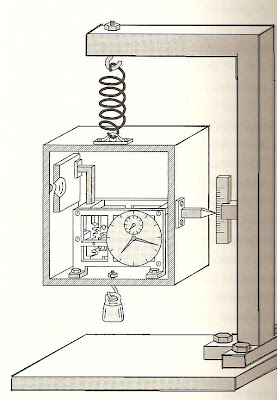## Saturday, December 11, 2010

### Quantum Acausal Determinism (3)

Einstein's "deterministic" weighing device which he contrived to attempt to outsmart Bohr.Most people outside physics are unaware there were seven Solvay Conferences in all, in the course of which the essential underpinnings and core interpretation of quantum mechanics was thrashed out. Throughout the series, the determinist Einstein attempted every which way he could to outsmart the quantum theorists, especially Neils Bohr. Once again, Einstein refused to accept that "God played dice with the universe" - meaning allowed indeterminism in quantum events and measurements to hold sway in his universe.

When we left off in the last instalment, we saw how (using the EPR) thought experiment, Einstein believed he finally had Bohr by the throat. Figuring Bohr might come up with some trick or sly explanation, Einstein went one better at the 6th Solvay Conference held in 1930, actually designing a device (see image) that he was convinced would have Bohr in tears trying to find a solution. (According to reports, it very nearly did, and a number of participants insisted Neils was in a state of "shock" believing there was no real solution.)

Using his hypothetical (purely on paper) device, Einstein wanted to put to rest once and for all the notion that quantum mechanics was complete, or was in any way a proper science. The mechanical device contrived by einstein was designed as a counter-example to the Heisenberg Uncertainty principle for energy and time which states:

dE (dt) >= h/ 2 pi

where dE is the uncertainty in energy, and dt the uncertainty in time.

In the device, a weight scale is found and one can see it when a door (front of box) opens, with the door controlled by a clock timer. Whenever the door flaps open, even for a split second, one photon escapes and the weight difference (between original box and after) can be computed using Einstein's mass-energy equation, e.g.: m = E/ c^2. Thus, the difference is taken:

Weight(before door opens) - weight (after - with 1 photon of mass m = E/ c^2 gone)

Thus, since the time for brief opening is known (dt) and the photon's mass can be deduced from the above weight difference, Einstein argued that one can in principle to determine to any level of accuracy both the photon's energy and time of passage without any need for the energy-time uncertainty principle.

In other words, the result could be found on a totally deterministic basis!

Bohr nearly went bonkers when he studied the device, and for hours worried there was no solution any maybe the wily determinist was correct after all. When Bohr did finally come upon the solution, he realized he'd hoisted the master on his own petard.

The thing Einstein overlooked was that his very act of weighing the box translated to observing its displacement (say, dr = r2 - r1) within the Earth's gravitational field. But in Einstein's general theory of relativity, he'd found that clocks actually do run slower in gravitational fields (a phenomenon called 'gravitational time dilation') In this case, for the Earth, one would have the fractional difference in proper time, as a fraction of time passage t:

dt/ t ~ GM(1/r1 - 1/r2) ~ g(dr)/ c^2

where G is the Newtonian graviational constant, M is the Earth's mass, and g is the acceleration of gravity (g = 980 cm/ sec^2 in cgs) and c = 3 x 10^10 cm/sec.

Let us say the box deflection (r2 - r1)was 0.001 mm = 0.0001cm, then:

dt/t ~ (980 cm/s^2)(10^-4 cm)/ (3 x 10^10 cm/sec)^2

dt/t ~ 10^-22

and for an interval say t = 0.01 sec, dt = 10^-22(0.01 sec) = 10^-24 sec

In other words, the observation would actually generate a time uncertainty of 10^-24 sec- and hence an uncertainty dE in the energy of the photon. In other words, after the displacement (r2 - r1) arising from the measurement, the the clock is in a gravitational field different from the original one. (The Energy uncertainty can meanwhile be computed from the Heisenberg energy -time relation to be dE~ 10^-10 J)

Quantum theory prevails again!

Einstein's challenges to Bohr in the aftermath were all kind of half-hearted and had nowhere near the intensity of his clock-door device work of art. Rather than join happily with other QM theorists at the last Solvay Conference in 1933 Einstein - the perpetual determinist- remained on the sidelines "feeling the same uneasiness as he had before".

He went to work separately, on a "unified field theory" while the quantum theory edifice was formulated to its present maturity without him.

Next: How Bohmian QM Re-Introduces determinism